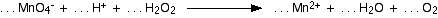Chemguide: Support for CIE A level Chemistry ``` ``` Learning outcome 24: Electrochemistry 24.2: Standard electrode potentials ``` ``` Learning outcome 24.2.7 This statement asks that you should be able to work out equations for redox equations from electron-half-equations. You may well be able to do this already. ``` ``` Read the page about writing ionic equations for redox reactions. You can ignore the link at the bottom of the page to a further section about working out half-equations under alkaline conditions. You are fairly unlikely to need this. For CIE purposes, it is more important that you should be able to combine two half-equations which you are given, rather than working them out from scratch. In the exam, you are quite likely to be given the half-equations you are likely to need. In one question, CIE asked you to balance the following equation:If you just try to balance this by playing around with the numbers, you will probably still be there an hour later, and still won't have got it right. You have to split these complicated redox equations into the two half-equations, and then combine them. Note:  Actually, if you do try to balance this equation by playing with the numbers, you stand a good chance of getting it wrong. It has been pointed out to me that there are an infinite number of ways of writing the numbers of hydrogen peroxide, water and oxygen molecules. Unfortunately, only one of these correctly represents the reacting proportions that you find in the lab - and that is the one that you get by working it out using the half-equations. Although all the other equations that you might find will balance properly, they are all wrong because they don't match what actually happens in the lab. You will find this worked out from scratch as Example 2 on the page you will get to from the link above. However, in this case, you don't actually have to work out the half-equations - you can find them from the Data section towards the end of the syllabus. Find the table of standard electrode potentials. Now find the half-equation for the change from MnO4- to Mn2+. This is shown as a reversible reaction, for reasons that you will discover in other parts of this section. The reaction you want is the forward reaction. Now find a half-equation that involves hydrogen peroxide and oxygen. In this case, you will find that the hydrogen peroxide is on the right-hand side of the equilibrium and oxygen on the left. In the reaction you are interested in, you have to go from hydrogen peroxide to oxygen, and so you need the reverse reaction. So you now have the two half-equations you need, and you can combine them as you will find on the equations page. This question was worth 1 mark, and the Examiner's Report said that only 20% of candidates could do it. That's not in the least surprising! Even if you knew what you were doing, it would take you several minutes to find and use the information to get the equation right. In Paper 4 (which is where this question comes from), you are working at the rate of almost exactly 1 mark per minute. Sometimes CIE examiners don't seem to have the faintest idea of what most A level candidates are capable of doing. ``` ``` Go to the Section 24 Menu . . . To return to the list of learning outcomes in Section 24 Go to the CIE Main Menu . . . To return to the list of all the CIE sections Go to Chemguide Main Menu . . . This will take you to the main part of Chemguide. © Jim Clark 2020